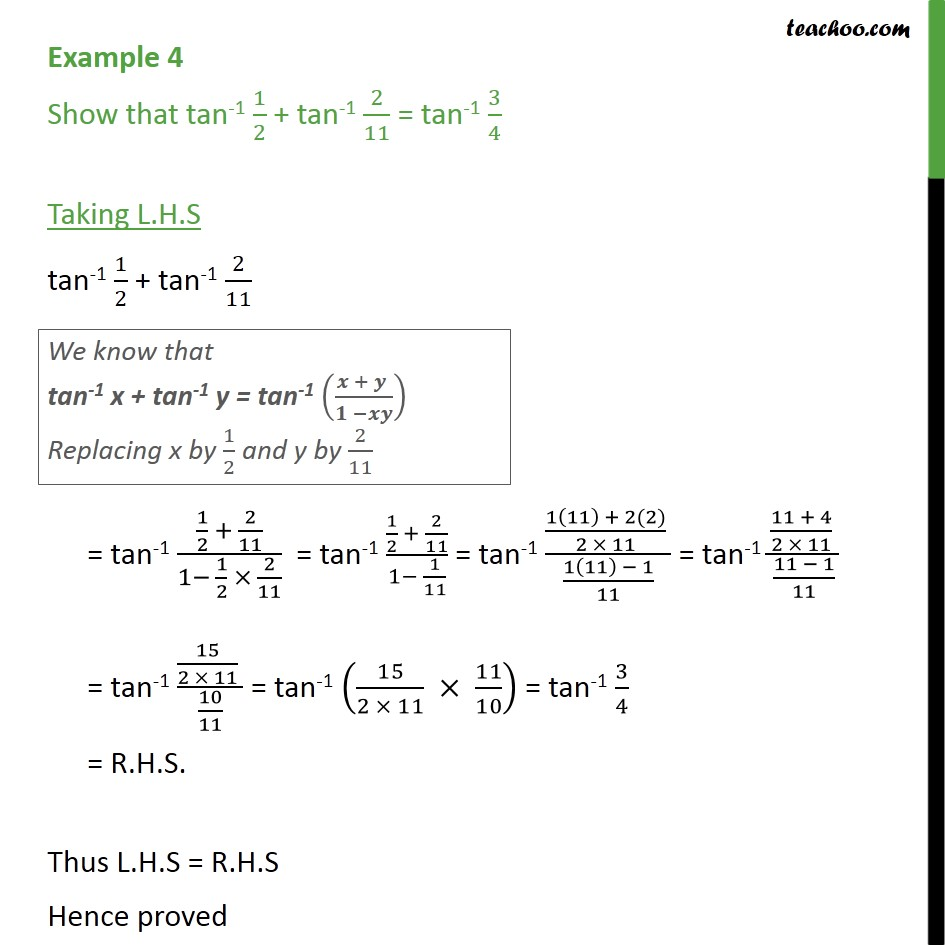Subscribe to our Youtube Channel - https://you.tube/teachoo

1. Chapter 2 Class 12 Inverse Trigonometric Functions
2. Concept wise
3. Formulae based

Transcript

Example 4 Show that tan-1 1/2 + tan-1 2/11 = tan-1 3/4 Taking L.H.S tan-1 1/2 + tan-1 2/11 = tan-1 (1/2 + 2/11)/(1 1/2 2/11) = tan-1 (1/2 + 2/11)/(1 1/11) = tan-1 ((1(11) + 2(2))/(2 11) )/((1(11) 1)/11) = tan-1 ((11 + 4)/(2 11) )/((11 1)/11) = tan-1 (15/(2 11) )/(10/11) = tan-1 (15/(2 11) 11/10) = tan-1 3/4 = R.H.S. Thus L.H.S = R.H.S Hence proved

Formulae based